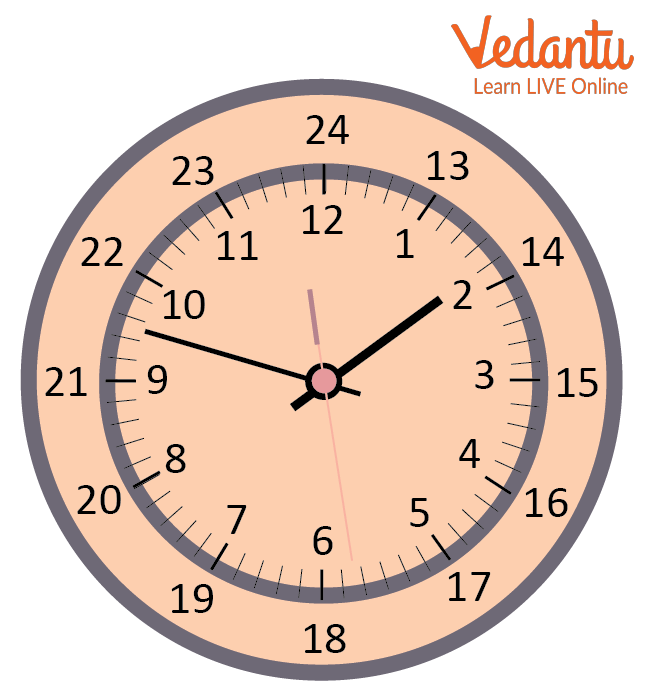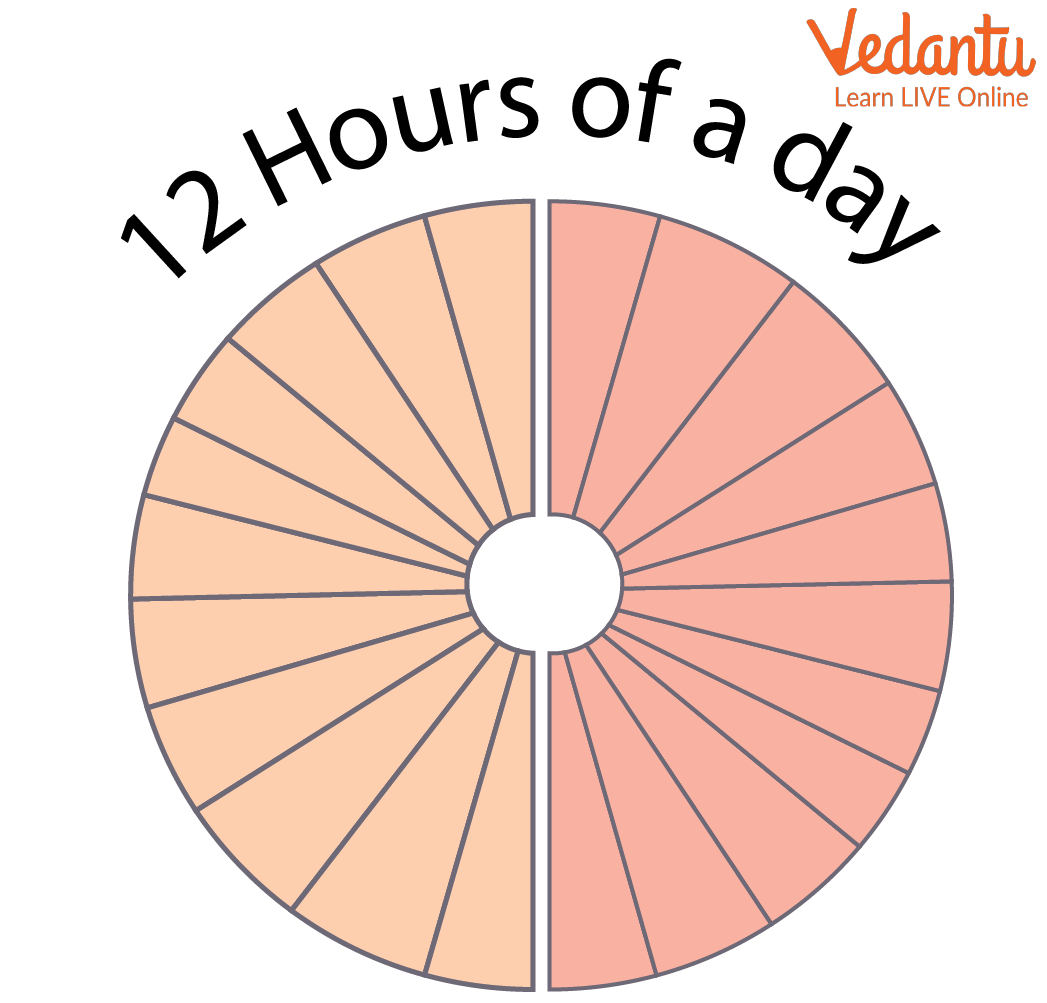Courses
Courses for Kids
Free study material
Free LIVE classes
More

# What Fraction of a Day is 12 HoursLIVE
Join Vedantu’s FREE Mastercalss

## Come Spend One Day with me: Fractions

Fractions are typically used to express the percent part of a number, as it is common in maths to show a percent by dividing this number by 100. For example, if 80% is equal to 0.80, then 80 must be divided by 100 to find out what number we are getting back. You must have seen fractions in your surroundings. Fractions are in clocks, pizza, or anything which is divided into equal parts. In this, you will be learning about “how to read fractions” and most importantly “what fraction of a day is 12 hours”.Time of the Clock Showing 12 hours and 24 hours

## What is the Meaning of Fraction?

A fraction is a number that is a whole number less than 1, divided by another whole number. In other words, it's one-half of the division. By definition, what is the meaning of fraction is that any time there are 3 or more parts in a fraction's name, you're representing an order quantity from the same group of numbers (1 through 9).

One part is called a fraction and the other part is called a multiplier. All common fractions can be reduced to these basic parts; as well as to many compound fractions. For example, if you want to know how to read fractions, one-half $\left(\dfrac{1}{2}\right)$ can be reduced to 1 and one-fourth $\left(\dfrac{1}{4}\right)$, or $\left(\dfrac{2}{3}\right)$ or $\left(\dfrac{7}{8}\right)$.

## Let's Look at 12 as a Fraction

The number 12, is the numerator (top part) of the fraction.

To get the denominator, or bottom part of the fraction, we just multiply the top part by 10. So you end up with 10 as your bottom number.

To know what is 12 as a fraction and to make a proper fraction, you round to 3 decimal places. One way to do this is to put your top number to the left of a line and then count by 10; in this case, that would be 2 spots up from 1 (1.2).

## What Fraction of a Day is 12 Hours?

A person would need to spend about 24 hours without sleep for there to be more than twelve on their body clock.

We've all been asked the question "What fraction of a day is 12 hours long?" and now you can know the answer! We're going to do some maths together to figure out what fraction of a day is 12 hours, so follow along with me if you want to know too.

There are 24 hours in a day, i.e 12 hours make the half day.Dividing the Total Hours of the Day into Fractions

Let’s see some of the solved examples and find the fraction solution based on the word problem.

## Solved Examples

Q1. Mrs. Brown has 24 apples. She ate $\left(\dfrac{1}{4}\right)$ of them. How many apples does she eat?

Solution:

Clearly, each group will contain 24 ÷ 4 = 6 apples.

Thus, $\left(\dfrac{1}{4}\right)$ of 24 is 6.

Mrs. Brown ate 6 apples.

Q2. Mrs. Brown has 24 apples. She ate 6 of them. Find the number of left-out apples.

Ans: Mrs. Brown ate 6 apples from 24 apples.

Number of left out apples = 24 – 6 = 18.

Q3. What fraction of a day is 8 hours?

Ans: We have,

One day = 24 hours.

Therefore, 8 hours = $\left(\dfrac{8}{24}\right)$ of a day.

Q4. Fran baked 12 lemon tarts for her son, Bob. He gobbled up 4 tarts. What fraction of lemon tarts did Bob eat?

Ans: No. of tarts Fran baked = 12

No. of tarts Bob ate = 4

Fraction of tarts Bob ate = $\left(\dfrac{4}{12}\right)$=$\left(\dfrac{1}{3}\right)$

Q5. Dylan has a total of 25 marbles. He gives 5 marbles to his sister, Jane. What fraction of marbles did Jane receive?

Ans: Total Number of marbles = 25 marbles

Dylan gives 5 marbles to Jane

The fraction of marbles did Jane received = $\left(\dfrac{5}{25}\right)$= $\left(\dfrac{1}{5}\right)$

## Practice Questions

Q1. Determine $\left(\dfrac{2}{3}\right)$ of a collection of 9 balls.

Ans: 6 balls.

Q2. Andrea has a packet of 20 biscuits. She gives $\left(\dfrac{1}{2}\right)$ of them to Andy and $\left(\dfrac{1}{4}\right)$ of them to Sally. The rest she keeps.

(i) How many biscuits does Andy get?

(ii) How many biscuits does Sally get?

(iii) How many biscuits does Andrea keep?

Ans:

(i) Andy gets 10 biscuits.

(ii) Sally gets 5 biscuits.

(iii) Andrea keeps 20 – 10 – 5 = 5 biscuits

Q3. What fraction of a day are 6 hours?

Ans: $\left(\dfrac{1}{4}\right)$

Q4. It takes Julia $\left(\dfrac{1}{2}\right)$ hour to wash, comb her hair, and put on her clothes, and $\left(\dfrac{1}{4}\right)$ hour to have her breakfast. How much time does it take Julia to be ready for school?

Ans: $\left(\dfrac{3}{4}\right)$ hours

Q5. Emily places 15 roses: 6 yellow roses and 9 red roses. What fraction of roses are red?

Ans: $\left(\dfrac{3}{5}\right)$ of roses are red.

## Summary

In the article first of all we have seen what the fraction is. A fraction is basically a part of a whole. As we know that in a day, there are a total of 24 hours. So, in an hour, it had become $\dfrac{1}{24}$ of a day. In this way, 12 hours is half of a day. Later on, we added some practice questions to deal with the concept of fractions. By solving these questions, you will get proper command over the basics of fractions.

Last updated date: 27th Sep 2023
Total views: 116.4k
Views today: 1.16k

## FAQs on What Fraction of a Day is 12 Hours

1. What does the word fraction signify in general?

The word 'fraction' in its basic form means "a part or share". It is similar to the word 'part' in that sense. It can also mean "an amount obtained by dividing a whole or a complete unit into parts". According to this meaning, the fraction is used for portions of an entire quantity.

2. What fraction of the earth's surface is covered by oceans?

Fractions are typically used to express the percent part of a number, as it is common in maths to show a percent by dividing this number by 100. Scientists sometimes use the word "fraction" to indicate proportions in units of measurement. For example, we can say that one-third of the Earth's surface is covered by oceans; another way to say this would be that two-thirds of the Earth's surface is not covered by oceans. In this situation, "third '' and "two-thirds" are fractions.

3. What are some of the real-world applications of fractions?

Fractions are used in many ways, They help in determining the parts of a whole. They are used in cooking for measurements, and they are used to make calculations. They are used to express scores in a test, time, offers in retail stores, etc. It is also used to divide pizzas.